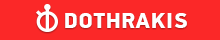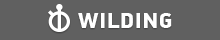GMAT Question of the Day - Daily to your Mailbox; hard ones only

 It is currently 26 Jun 2019, 11:46GMAT Club Daily Prep

Thank you for using the timer - this advanced tool can estimate your performance and suggest more practice questions. We have subscribed you to Daily Prep Questions via email.

Customized
for You

we will pick new questions that match your level based on your Timer History

Track

every week, we’ll send you an estimated GMAT score based on your performance

Practice
Pays

we will pick new questions that match your level based on your Timer History

Not interested in getting valuable practice questions and articles delivered to your email? No problem, unsubscribe here.Show Tags

100:00

Difficulty:15% (low)

Question Stats:88% (02:25) correct12% (02:43) wrongbased on 33 sessions

HideShow timer StatisticsHow many nails did Rudy buy if he purchased them at a price of $0.25 per four nails, sold them at$0.22 per three nails, and made a profit of $2.60? A. 300 B. 240 C. 180 D. 160 E. 120 Originally posted by Nums99 on 12 Jan 2019, 01:42. Last edited by Bunuel on 12 Jan 2019, 03:25, edited 1 time in total. Renamed the topic, edited the question, moved to PS and added the OA. Senior ManagerD Joined: 15 Jan 2018 Posts: 371 Concentration: General Management, Finance GMAT 1: 720 Q50 V37Re: How many nails did Rudy buy if he purchased them at a price of$0.25  [#permalink]

Show Tags

1
Hi there,

Here's the solution.

Let the number of nails Rudy purchased be x.

Rudy purchased nails at a price of $0.25 per four nails. So, Cost Price of 4 nails =$0.25
Cost Price of 1 nail = = $0.25/4 Cost price of x nails = ($0.25/4)x

Also given that Rudy sold nails at $0.22 per three nails. So, Selling Price of 3 nails =$0.22
Selling Price of 1 nail = = $0.22/3 Selling price of x nails = ($0.22/3)x

Also, Rudy made a profit of $2.60. Profit = Selling Price - Cost Price$2.60 = ($0.22/3)x - ($0.25/4)x = x(0.88 - 0.75)/12
$2.60 = x(0.13)/12 x = 240 Hence, the the number of nails Rudy purchased is 240. Therefore, the Correct Answer is Option B. 240 _________________ New to GMAT Club or overwhelmed with so many resources? Follow the GMAT Club Study Plan! Not happy with your GMAT score? Retaking GMAT Strategies! Game of Timers - Join the Competition to Win Prizes Senior ManagerD Status: Manager Joined: 27 Oct 2018 Posts: 365 Location: Egypt Concentration: Strategy, International Business GPA: 3.67 WE: Pharmaceuticals (Health Care) Re: How many nails did Rudy buy if he purchased them at a price of$0.25  [#permalink]

Show Tags

Nums99 wrote:
How many nails did Rudy buy if he purchased them at a price of $0.25 per four nails, sold them at$0.22 per three nails, and made a profit of $2.60? A. 300 B. 240 C. 180 D. 160 E. 120 Profit = Sales - Cost $$2.6 = \frac{0.22}{3}n - \frac{0.25}{4}n = \frac{0.88n}{12} - \frac{0.75n}{12}$$ $$\frac{13}{5} = \frac{0.13n}{12} = \frac{13n}{1200}$$ $$n = \frac{13*1200}{13*5} = 240$$ B _________________ RC ModeratorV Joined: 24 Aug 2016 Posts: 802 Location: Canada Concentration: Entrepreneurship, Operations GMAT 1: 630 Q48 V28GMAT 2: 540 Q49 V16Re: How many nails did Rudy buy if he purchased them at a price of$0.25  [#permalink]

Show Tags

As we are dealing with two numbers 3 & 4 .... lets take the LCM of them i.e.,12 .
Cost price for 12 nails = $0.25*12/4=$0.75 & Selling price for 12 nails = $0.22*12/3=$0.88
Profit made per 12 nails = $0.88 -$0.75 = $0.13 So total '12 units' sold =$2.60/$0.13 =20 Total nails sold = 20*12 = 240 .... Thus Ans would be option B. _________________ Please let me know if I am going in wrong direction. Thanks in appreciation. DirectorV Joined: 12 Feb 2015 Posts: 863 Re: How many nails did Rudy buy if he purchased them at a price of$0.25  [#permalink]

Show Tags

Nums99 wrote:
How many nails did Rudy buy if he purchased them at a price of $0.25 per four nails, sold them at$0.22 per three nails, and made a profit of $2.60? A. 300 B. 240 C. 180 D. 160 E. 120 LCM approach is good in such questions:- Rudy purchased nails at a price of$0.25 per four nails, or $0.75 per 12 nails sold them at$0.22 per three nails, or $0.88 per 12 nails and made a profit of$2.60 (total) or $(0.88-0.75) =$ 0.13 per 12 nails

(2.60 * 12)/0.13 = 240 nails (Ans)
_________________
"Please hit+1 Kudos if you like this post"_________________
Manish"Only I can change my life. No one can do it for me"
DirectorV
Joined: 04 Dec 2015
Posts: 740
Location: India
Concentration: Technology, Strategy
WE: Information Technology (Consulting)
How many nails did Rudy buy if he purchased them at a price of $0.25 [#permalink] Show Tags Nums99 wrote: How many nails did Rudy buy if he purchased them at a price of$0.25 per four nails, sold them at $0.22 per three nails, and made a profit of$2.60?

A. 300
B. 240
C. 180
D. 160
E. 120

Cost Price of $$4$$ nails $$=  0.25$$

Cost Price of $$3$$ nails $$= \frac{0.25*3}{4} =  0.1875$$

Selling Price of $$3$$ nails $$= 0.22$$

Profit on $$3$$ nails $$= 0.22 - 0.1875 =  0.0325$$

Let $$"x"$$ be total number of nails to make profit of $$2.60$$.

$$\frac{0.0325}{3} = \frac{2.60}{x}$$

$$0.0325*x =3*2.60$$

$$x = \frac{3*2.60}{0.0325} = 240$$

Target Test Prep RepresentativeD
Status: Founder & CEO
Affiliations: Target Test Prep
Joined: 14 Oct 2015
Posts: 6680
Location: United States (CA)
Re: How many nails did Rudy buy if he purchased them at a price of $0.25 [#permalink] Show Tags Nums99 wrote: How many nails did Rudy buy if he purchased them at a price of$0.25 per four nails, sold them at $0.22 per three nails, and made a profit of$2.60?

A. 300
B. 240
C. 180
D. 160
E. 120

We can let n = the number of nails and create the equation:

(0.22/3)n - (0.25/4)n = 2.6

Multiplying by 12, we have:

0.22 x 4n - 0.25 x 3n = 31.2

0.88n - 0.75n = 31.2

0.13n = 31.2

n = 240

_________________

Scott Woodbury-Stewart

Founder and CEO

Scott@TargetTestPrep.com

See why Target Test Prep is the top rated GMAT quant course on GMAT Club. Read Our Reviews

If you find one of my posts helpful, please take a moment to click on the "Kudos" button.Re: How many nails did Rudy buy if he purchased them at a price of $0.25 [#permalink] 02 Mar 2019, 10:08 Display posts from previous: Sort by How many nails did Rudy buy if he purchased them at a price of$0.25